SSC CGL Previous Year Questions: Percentage - 2

# SSC CGL Previous Year Questions: Percentage - 2 - SSC CGL

Test Description

## 40 Questions MCQ Test SSC CGL (Tier - 1) - Previous Year Papers (Topic Wise) - SSC CGL Previous Year Questions: Percentage - 2

SSC CGL Previous Year Questions: Percentage - 2 for SSC CGL 2023 is part of SSC CGL (Tier - 1) - Previous Year Papers (Topic Wise) preparation. The SSC CGL Previous Year Questions: Percentage - 2 questions and answers have been prepared according to the SSC CGL exam syllabus.The SSC CGL Previous Year Questions: Percentage - 2 MCQs are made for SSC CGL 2023 Exam. Find important definitions, questions, notes, meanings, examples, exercises, MCQs and online tests for SSC CGL Previous Year Questions: Percentage - 2 below.
Solutions of SSC CGL Previous Year Questions: Percentage - 2 questions in English are available as part of our SSC CGL (Tier - 1) - Previous Year Papers (Topic Wise) for SSC CGL & SSC CGL Previous Year Questions: Percentage - 2 solutions in Hindi for SSC CGL (Tier - 1) - Previous Year Papers (Topic Wise) course. Download more important topics, notes, lectures and mock test series for SSC CGL Exam by signing up for free. Attempt SSC CGL Previous Year Questions: Percentage - 2 | 40 questions in 41 minutes | Mock test for SSC CGL preparation | Free important questions MCQ to study SSC CGL (Tier - 1) - Previous Year Papers (Topic Wise) for SSC CGL Exam | Download free PDF with solutions
 1 Crore+ students have signed up on EduRev. Have you?
SSC CGL Previous Year Questions: Percentage - 2 - Question 1

### A number is increased by 15% and then decreased by 25% and the number becomes 22 less than the original number. The original number is    (SSC Sub. Ins. 2016)

Detailed Solution for SSC CGL Previous Year Questions: Percentage - 2 - Question 1

Let the number be 100
Number increased by 15% = 115
Number decreased by 25%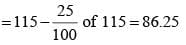According to question,
(100 – 86.25) unit → 22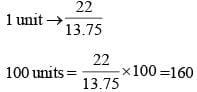Hence, original number is 160.

SSC CGL Previous Year Questions: Percentage - 2 - Question 2

### In a motor of 120 machine parts, 5% parts were defective. In another motor of 80 machine parts, 10% parts were defective. For the two motors considered together, the percentage of defective machine parts were    (SSC CGL 2nd Sit. 2016)

Detailed Solution for SSC CGL Previous Year Questions: Percentage - 2 - Question 2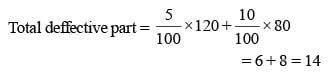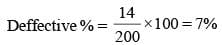SSC CGL Previous Year Questions: Percentage - 2 - Question 3

### The price of rice has increased by 60%. In order to restore the original price, the new price must be reduced by    (SSC CGL 2nd Sit. 2016)

Detailed Solution for SSC CGL Previous Year Questions: Percentage - 2 - Question 3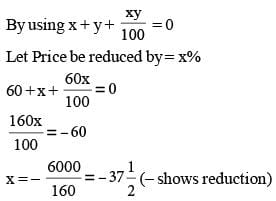SSC CGL Previous Year Questions: Percentage - 2 - Question 4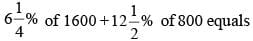(SSC CGL 2nd Sit. 2016)

Detailed Solution for SSC CGL Previous Year Questions: Percentage - 2 - Question 4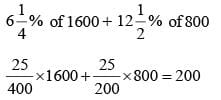SSC CGL Previous Year Questions: Percentage - 2 - Question 5

If 35% of A’s income is equal to 25% of B’s income, then the ratio of A’s income to B’s income is    (SSC CGL 1st Sit. 2016)

Detailed Solution for SSC CGL Previous Year Questions: Percentage - 2 - Question 5

35 % A’s Salary = 25% of B’s Salary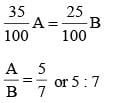SSC CGL Previous Year Questions: Percentage - 2 - Question 6

A basket contains 300 mangoes. 75 mangoes were distributed among some students. Find the percentage of mangoes left in the basket    (SSC CGL 1st Sit. 2016)

Detailed Solution for SSC CGL Previous Year Questions: Percentage - 2 - Question 6

Total mango = 300
Distribution = 75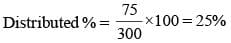Percentage of mangoes left in the basket = 75%

SSC CGL Previous Year Questions: Percentage - 2 - Question 7

In an examination, a student must get 36% marks to pass. A student who gets 190 marks failed by 35 marks. The total marks in that examination is:    (SSC CGL 1st Sit. 2015)

Detailed Solution for SSC CGL Previous Year Questions: Percentage - 2 - Question 7

Let total mark of Examination be x.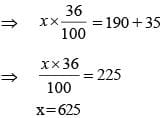SSC CGL Previous Year Questions: Percentage - 2 - Question 8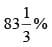of ₹90 is equal to 60% of?    (SSC CHSL 2015)

Detailed Solution for SSC CGL Previous Year Questions: Percentage - 2 - Question 8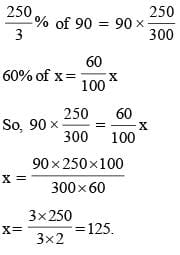SSC CGL Previous Year Questions: Percentage - 2 - Question 9

A number is increased by x%, to get back to the original number, it is to be reduced by:    (SSC Sub. Ins. 2015)

Detailed Solution for SSC CGL Previous Year Questions: Percentage - 2 - Question 9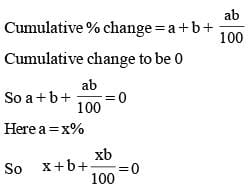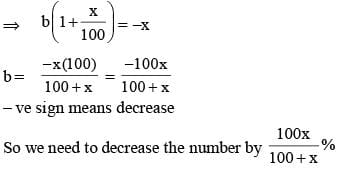SSC CGL Previous Year Questions: Percentage - 2 - Question 10

The height of a triangle is increased by 10%. To retain the original area of the triangle, its corresponding base must be decreased by:    (SSC Sub. Ins. 2015)

Detailed Solution for SSC CGL Previous Year Questions: Percentage - 2 - Question 10

Let base = b, Height = h,

So, Area = (b × h)/2So, Reduced base = [(½ b × h)/1.1h] × 2 = 10b/11

Percent reduction in base = [(b – 10b/11)/b] × 100

∴ Percent change in base = (100/11)%

SSC CGL Previous Year Questions: Percentage - 2 - Question 11

The monthly salaries of A and B together amount to ₹ 40,000. A spends 85% of his salary and B, 95% of his salary. If now their savings are the same, then the salary (in ₹) of A is    (SSC CGL 2014)

Detailed Solution for SSC CGL Previous Year Questions: Percentage - 2 - Question 11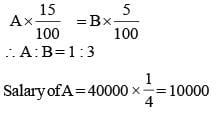SSC CGL Previous Year Questions: Percentage - 2 - Question 12

1% of 1% of 25% of 1000 is    (SSC CHSL 2014)

Detailed Solution for SSC CGL Previous Year Questions: Percentage - 2 - Question 12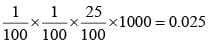SSC CGL Previous Year Questions: Percentage - 2 - Question 13

If 40% of 4/5 of 3/4 of a number is 48, then what is 1% of the same number?    (SSC Sub. Ins. 2014)

Detailed Solution for SSC CGL Previous Year Questions: Percentage - 2 - Question 13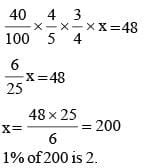SSC CGL Previous Year Questions: Percentage - 2 - Question 14

In an examination 75% candidates passed in English and 60% passed in Mathematics. 25% failed in both and 240 passed the examination. Find the total number of candidates.   (SSC Sub. Ins. 2014)

Detailed Solution for SSC CGL Previous Year Questions: Percentage - 2 - Question 14

Let the total number of students be x.
Let A and B represent the sets of students who passed in English and Mathematics respectively.
Then, number of students passed in one or both the subjects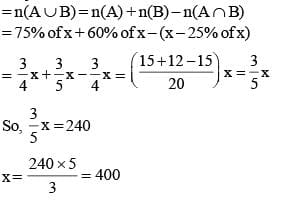SSC CGL Previous Year Questions: Percentage - 2 - Question 15

A sales representative will receive a 15% commission on a sale of ₹ 2,800. If he has already received an advance of ₹ 150 on that commission, the remaining amount of commission is    (SSC Multitasking 2014)

Detailed Solution for SSC CGL Previous Year Questions: Percentage - 2 - Question 15

Sales representative will  receive total amount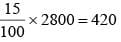Remaining amount = 420 – 150 = 270

SSC CGL Previous Year Questions: Percentage - 2 - Question 16

In a school, 10% of number of girls is equal to 20th of number of boys. Ratio between the number of boys to number of girls is    (SSC CGL 2nd Sit. 2013)

Detailed Solution for SSC CGL Previous Year Questions: Percentage - 2 - Question 16

If boys = x and girls = y, then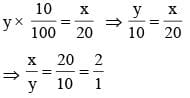SSC CGL Previous Year Questions: Percentage - 2 - Question 17

Rakesh got 273 marks in an examination and scored 5% more than the pass %. If Lokesh got 312 marks, then by what % above the pass mark did he pass the examination?    (SSC CGL 2nd Sit. 2013)

Detailed Solution for SSC CGL Previous Year Questions: Percentage - 2 - Question 17

Let passing marks be represented by p.
p × 1.05 = 273
p = 260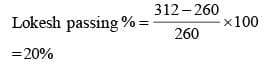SSC CGL Previous Year Questions: Percentage - 2 - Question 18

A box has 100 blue balls, 50 red balls, 50 black ball. 25% of blue balls and 50% of red balls are taken away. percentage of black balls at present is    (SSC CGL 2nd Sit. 2013)

Detailed Solution for SSC CGL Previous Year Questions: Percentage - 2 - Question 18

After taking away respective balls,
Number of balls in the box = 75 + 25 + 50 = 150
∴ Percentage of black balls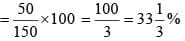SSC CGL Previous Year Questions: Percentage - 2 - Question 19

Given A is 50% larger than C and B is 25% large than C, then A is what percent larger than B?    (SSC CGL 1st Sit. 2013)

Detailed Solution for SSC CGL Previous Year Questions: Percentage - 2 - Question 19

C = 100
A = 150
B = 125
A is larger than B by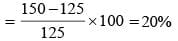SSC CGL Previous Year Questions: Percentage - 2 - Question 20

A number when reduced by 10% gives 30. The number is   (SSC Multitasking 2013)

Detailed Solution for SSC CGL Previous Year Questions: Percentage - 2 - Question 20

Let the number is x.
According to question
x – 10% of  x = 30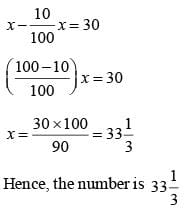SSC CGL Previous Year Questions: Percentage - 2 - Question 21

Two persons contested an election of Parliament. The winning candidate secured 57% of the total votes polled and won by a majority of 42,000 votes. The number of total votes polled is    (SSC Multitasking 2013)

Detailed Solution for SSC CGL Previous Year Questions: Percentage - 2 - Question 21

Let x be the total number of polled votes.
Then, (57–43)% of x = 42000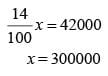SSC CGL Previous Year Questions: Percentage - 2 - Question 22

A certain amount of money is divided among x, y and z. If x receives 25% more than y and y receives 25% less than z, then x : y : z is equal to   (SSC Multitasking 2013)

Detailed Solution for SSC CGL Previous Year Questions: Percentage - 2 - Question 22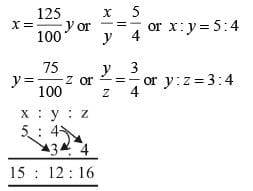Then, x : y : z is equal to 15 : 12 : 16

SSC CGL Previous Year Questions: Percentage - 2 - Question 23

In a big garden 60% of the trees are coconut trees, 25% of the number of coconut trees are mango trees and 20% of the number of mango trees are apple trees. If the number of apple trees are 1500. then the number of trees in the garden is:          (SSC Sub. Ins. 2013)

Detailed Solution for SSC CGL Previous Year Questions: Percentage - 2 - Question 23

If the number of trees in the garden be x, then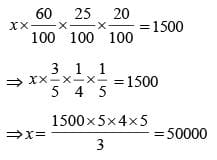SSC CGL Previous Year Questions: Percentage - 2 - Question 24

If 125% of x is 100, then x is:    (SSC CHSL 2012)

Detailed Solution for SSC CGL Previous Year Questions: Percentage - 2 - Question 24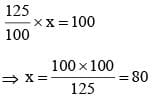SSC CGL Previous Year Questions: Percentage - 2 - Question 25

A team played 40 games in a season and won in 24 of them. What percent of games played did the team win?    (SSC CHSL 2012)

Detailed Solution for SSC CGL Previous Year Questions: Percentage - 2 - Question 25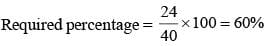SSC CGL Previous Year Questions: Percentage - 2 - Question 26

72% of the students of a certain class took Biology and 44% took Mathematics. If each student took Biology or Mathematics and 40 took both, the total number of students in the class was     (SSC Sub. Ins. 2012)

Detailed Solution for SSC CGL Previous Year Questions: Percentage - 2 - Question 26

Let the total number of students in the class be x.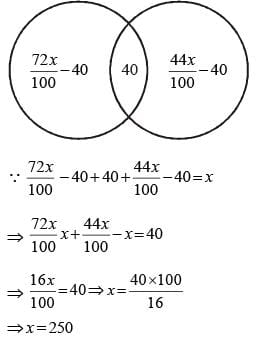SSC CGL Previous Year Questions: Percentage - 2 - Question 27

The salary of an employee increases every year in the month of July by 10%. If his salary in May 2000 was ₹ 15,000, his salary in October 2001 was    (SSC Sub. Ins. 2012)

Detailed Solution for SSC CGL Previous Year Questions: Percentage - 2 - Question 27

Salary in May 2000 = ₹ 15000
Salary in July 2000 ⇒ 15000 + 10% of 15000 = ₹16500
Salary in October 2001 = 16500 + 10% of 16500 = ₹ 18150

SSC CGL Previous Year Questions: Percentage - 2 - Question 28

The number of seats in an auditorium is increased by 25%. The price of a ticket is also increased by 12%. Then the increase in revenue collection will be    (SSC CGL 1st Sit. 2012)

Detailed Solution for SSC CGL Previous Year Questions: Percentage - 2 - Question 28

Required increase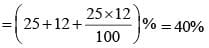SSC CGL Previous Year Questions: Percentage - 2 - Question 29

The number 20% more than 80 is   (SSC CGL 2012)

Detailed Solution for SSC CGL Previous Year Questions: Percentage - 2 - Question 29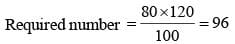SSC CGL Previous Year Questions: Percentage - 2 - Question 30

The population of a town is 15000. If the number of males increases by 8% and that of females by 10%, then the population would increase to 16300. Find the number of females in the town.    (SSC CGL 2012)

Detailed Solution for SSC CGL Previous Year Questions: Percentage - 2 - Question 30

If the number of females be x, then, number of males = 15000 – x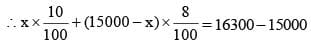⇒ 10x + 120000 – 8x = 1300 × 100
⇒ 2x = 130000 – 120000 = 10000
⇒ x = 5000

SSC CGL Previous Year Questions: Percentage - 2 - Question 31

First and second numbers are less than a third number by 30% and 37% respectively. The second number is less than the first by    (SSC CGL 2011)

Detailed Solution for SSC CGL Previous Year Questions: Percentage - 2 - Question 31

Let the third number = 100
First number = 70
Second number = 63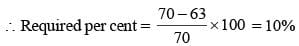SSC CGL Previous Year Questions: Percentage - 2 - Question 32

If 90% of  A = 30% of B and B = x% of A, then the value of x is   (SSC CGL 2011)

Detailed Solution for SSC CGL Previous Year Questions: Percentage - 2 - Question 32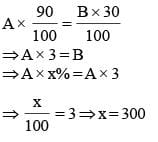SSC CGL Previous Year Questions: Percentage - 2 - Question 33

If 30% of A is added to 40% of B, the answer is 80% of B. What percentage of A is B?   (SSC CGL 2011)

Detailed Solution for SSC CGL Previous Year Questions: Percentage - 2 - Question 33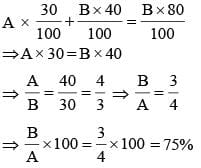SSC CGL Previous Year Questions: Percentage - 2 - Question 34

If 90% of A = 30% of B and B = 2x % of A, then the value of x is    (SSC CGL 1st Sit. 2011)

Detailed Solution for SSC CGL Previous Year Questions: Percentage - 2 - Question 34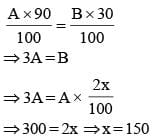SSC CGL Previous Year Questions: Percentage - 2 - Question 35

If an electricity bill is paid before due date, one gets a reduction of 4% on the amount of the bill. By paying the bill before due date a person got a reduction of ₹ 13. The amount of his electricity bill was    (SSC CGL 2nd Sit. 2010)

Detailed Solution for SSC CGL Previous Year Questions: Percentage - 2 - Question 35

Let the amount of the bill be ₹ x.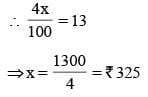SSC CGL Previous Year Questions: Percentage - 2 - Question 36

If A's income is 25% less than B's income, by how much percent is B's income more than that of A?    (SSC CGL 2nd Sit. 2010)

Detailed Solution for SSC CGL Previous Year Questions: Percentage - 2 - Question 36

Required percentage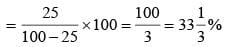SSC CGL Previous Year Questions: Percentage - 2 - Question 37

Two successive price increases of 10% and 10% of an article are equivalent to a single price increase of    (SSC CGL 2nd Sit. 2010)

Detailed Solution for SSC CGL Previous Year Questions: Percentage - 2 - Question 37

Single equivalent percentage increase in price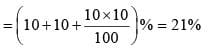SSC CGL Previous Year Questions: Percentage - 2 - Question 38

If 60% of A = 3/4 of B, then A : B is    (SSC CGL 1st Sit. 2010)

Detailed Solution for SSC CGL Previous Year Questions: Percentage - 2 - Question 38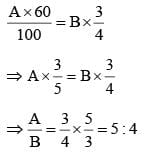SSC CGL Previous Year Questions: Percentage - 2 - Question 39

1.14 expressed as a percent of 1.9 is    (SSC CGL 1st Sit. 2010)

Detailed Solution for SSC CGL Previous Year Questions: Percentage - 2 - Question 39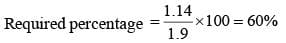SSC CGL Previous Year Questions: Percentage - 2 - Question 40

If A's income is 50% less than that of B's, then B's income is what per cent more than that of A?    (SSC CGL 1st Sit. 2010)

Detailed Solution for SSC CGL Previous Year Questions: Percentage - 2 - Question 40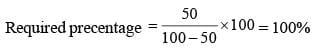## SSC CGL (Tier - 1) - Previous Year Papers (Topic Wise)

250 tests
Information about SSC CGL Previous Year Questions: Percentage - 2 Page
In this test you can find the Exam questions for SSC CGL Previous Year Questions: Percentage - 2 solved & explained in the simplest way possible. Besides giving Questions and answers for SSC CGL Previous Year Questions: Percentage - 2, EduRev gives you an ample number of Online tests for practice

250 tests